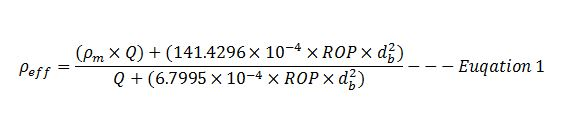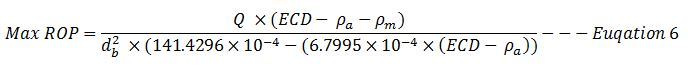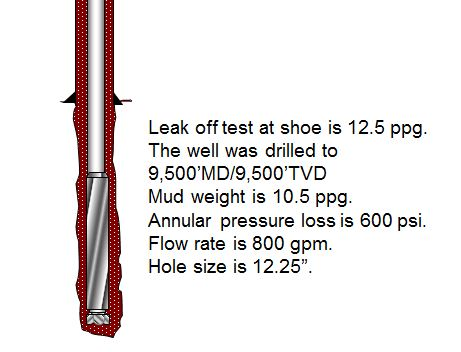# Maximum ROP Before Fracture Formation

In this topic, we will apply the effective mud density formula to determine maximum ROP before fracturing formation.Figure 1 – Max ROP before Fracturing Formation

These two equations that will be used to determine the maximum ROP are listed below;

Effective mud density due to cuttings in the hole can be determined by the empirical equation below;Where;

ρeff is effective mud density in ppg.

ρm is mud density in ppg.

Q is flow rate in gpm.

ROP is rate of penetration in fph.

db is wellbore diameter or bit diameter in inch.

Equivalent Circulating Density (ECD) consists of three components given by the equation below;

Equivalent Circulating Density (ECD) = ρm + ρc + ρa — Equation 2

Where;

ρm is mud density in ppg.

ρc is cutting density in ppg.

ρa is annular pressure loss in ppg.

How To Apply These Two Concepts

Formation will be fractured if the ECD while drilling is more than fracture gradient. Additionally, besides mud weight (ρm), cutting density and annular pressure loss due to hydraulic contributes to ECD.

The ECD can be described in term of effective density and annular pressure as listed below;

Equivalent Circulating Density (ECD) = ρm + ρc + ρa — Equation 2

ρeff  = ρm + ρc — Equation 3

Equivalent Circulating Density (ECD) = ρeff + ρ — Equation 4

Equivalent Circulating Density (ECD) = Fracture Gradient (FG) — Equation 5

With those relationships above, we can derive the maximum ROP into the following formulas.Example – Determine the maximum ROP for this well.Figure 2 – Well Information

Leak off test at shoe is 12.5 ppg.

The well was drilled to 9,500’MD/9,500’TVD

Mud weight is 10.5 ppg.

Annular pressure loss is 600 psi.

Flow rate is 800 gpm.

Hole size is 12.25”.

Solution

ECD should not exceed the leak off test value; therefore ECD is equal to 12.5 ppg.

Annular pressure loss (ρa) = 600 ÷ (0.052 x 9,500) = 1.21 ppgMax ROP = 647.2 fph

Share the joy